# Precalculus : Solve Absolute Value Inequalities

## Example Questions

2 Next →

### Example Question #41 : Inequalities And Linear Programming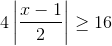Possible Answers: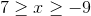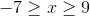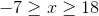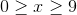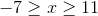Correct answer:Explanation:

To solve absolute value inequalities, you have to write it two different ways. But first, divide out the 4 on both sides so that there is just the absolute value on the left side. Then, write it normally, as you see it: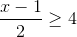and then flip the side and make the right side negative: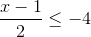. Then, solve each one. Your answers are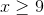and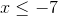.

### Example Question #42 : Inequalities And Linear Programming

Solve the following: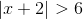Possible Answers: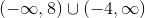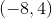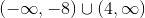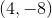Correct answer:Explanation:

To solve absolute value inequalities create two functions.

Simply remember that when solving inequalities with absolute values, you keep one the same and then flip the sign and inequality on the other.

Thus,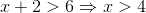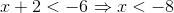Then, you must write it in interval notation.

Thus,2 Next →

### All Precalculus Resources